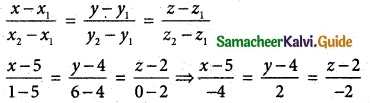Tamilnadu State Board New Syllabus Samacheer Kalvi 12th Maths Guide Pdf Chapter 6 Applications of Vector Algebra Ex 6.5 Textbook Questions and Answers, Notes.

## Tamilnadu Samacheer Kalvi 12th Maths Solutions Chapter 6 Applications of Vector Algebra Ex 6.5

Question 1.
Find the parametric form of vector equation and Cartesian equations of straight line passing through (5, 2, 8) and is perpendicular to the straight lines
$$\overline { r }$$ = ($$\hat { i }$$ + $$\hat { j }$$ – $$\hat { k }$$) + s(2$$\hat { i }$$ – 2$$\hat { j }$$ + $$\hat { k }$$) and
$$\overline { r }$$ = (2$$\hat { i }$$ – $$\hat { j }$$ – 3$$\hat { k }$$) + t($$\hat { i }$$ + 2$$\hat { j }$$ + 2$$\hat { k }$$).
Solution:
Given points (5, 2, 8)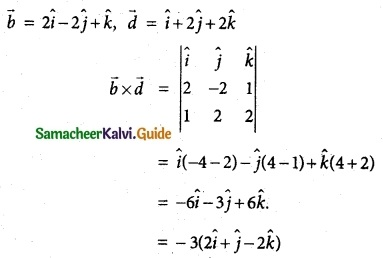2$$\hat { i }$$ + $$\hat { j }$$ + 3$$\hat { k }$$ is a vector perpendicular to both the given straight lines it passes through (5, 2, 8)
The equation is $$\overline { r }$$ = (5$$\hat { i }$$ + 2$$\hat { j }$$ + 8$$\hat { k }$$) + t(2$$\hat { i }$$ + $$\hat { j }$$ – 2$$\hat { k }$$)
Cartesian form = $$\frac { x-5 }{ 2 }$$ = $$\frac { y-2 }{ 1 }$$ = $$\frac { z-8 }{ -2 }$$Question 2.
Show that the lines
$$\overline { r }$$ = (6$$\hat { i }$$ + $$\hat { j }$$ + 2$$\hat { k }$$) + s($$\hat { i }$$ + 2$$\hat { j }$$ – 3$$\hat { k }$$) and
$$\overline { r }$$ = (3$$\hat { i }$$ + 2$$\hat { j }$$ – 2$$\hat { k }$$) + t(2$$\hat { i }$$ + 4$$\hat { j }$$ – 5$$\hat { k }$$) are skew lines and hence find the shortest distance between them.
Solution: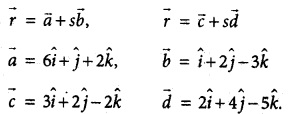$$\overline { b }$$ is not a scalar multiple of $$\overline { d }$$
∴ They are not parallel.
∴ The given lines are skew lines.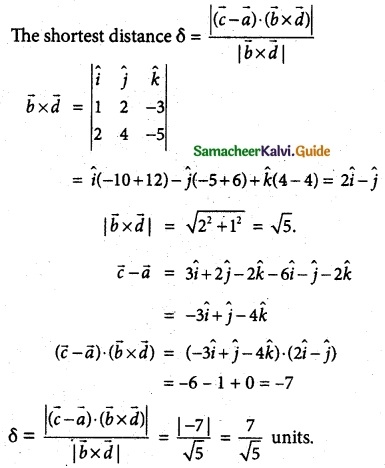Question 3.
If the two lines $$\frac { x-1 }{ 2 }$$ = $$\frac { y+1 }{ 3 }$$ = $$\frac { z-1 }{ 4 }$$ and $$\frac { x-3 }{ 1 }$$ = $$\frac { y-m }{ 2 }$$ = z intersect at a point, find the value of m.
Solution:
(x1, y1, z1) = (1, -1, 1), (x2, y2, z2) = (3, m, 0)
(1, 2, b3) = (2, 3, 4), (d1, d2, d3) = (1, 2, 1).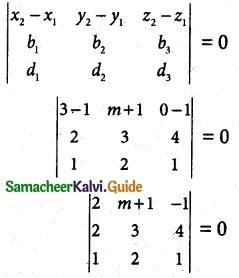2(3 – 8) – (m + 1) (2 – 4) – 1(4 – 3) = 0
-10 – (m + 1) (-2) – 1(1) = 0
-10 + 2m + 2 – 1 = 0
2m – 9 = 0
2m = 9
m = $$\frac { 9 }{ 2 }$$

Question 4.
Show that the lines $$\frac { x-3 }{ 3 }$$ = $$\frac { y-3 }{ -1 }$$, z – 1 = 0 and $$\frac { x-6 }{ 2 }$$ = $$\frac { z-1 }{ 3 }$$, y – 2 = 0 intersect. Also find the point of intersection.
Solution: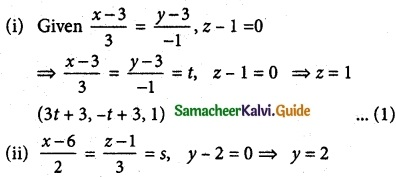(2s + 6, 2, 3s + 1) ……… (2)
(1) = (2)
((3t + 3), -t + 3, 1) = (2s + 6, 2, 3s + 1)
Compare on both sides
-t + 3 = 2; 3s + 1 = 1
t = 3 – 2; 3s = 0
t = 1; s = 0
(1) ⇒ Point of intersect (6, 2, 1)Question 5.
Show that the straight lines x + 1 = 2y = -12z and x = y + 2 = 6z – 6 are skew and hence find the shortest distance between them.
Solution:
Given x + 1 = 2y = -12z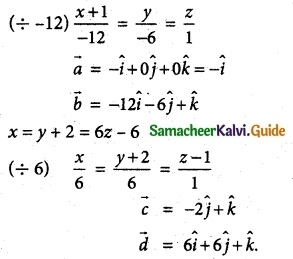$$\overline { b }$$ is not a scalar multiple of $$\overline { d }$$. So, the two vectors are not parallel.
∴ The given lines are skew lines.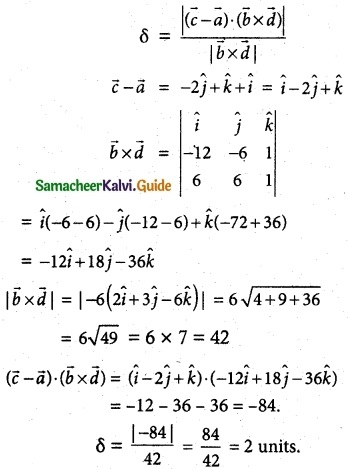Question 6.
Find the parametric form of vector equation of the straight line passing through (-1, 2, 1) and parallel to the straight line
$$\overline { r }$$ = (2$$\hat { i }$$ + 3$$\hat { j }$$ – $$\hat { k }$$) + t($$\hat { i }$$ – 2$$\hat { j }$$ + $$\hat { k }$$) and hence find the shortest distance between the lines.
Solution: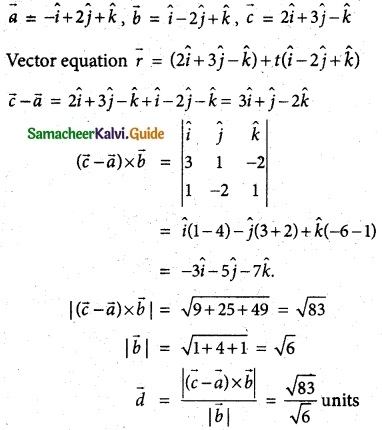Question 7.
Find the foot of the perpendicular drawn: from the point (5, 4, 2) to the line $$\frac { x+1 }{ 2 }$$ = $$\frac { y-3 }{ 3 }$$ = $$\frac { z-1 }{ -1 }$$. Also, find the equation of the perpendicular.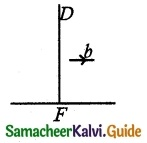Solution:
$$\overline { r }$$ = $$\overline { a }$$ + t$$\overline { b }$$
$$\overline { a }$$ = -i + 3j + k, $$\overline { b }$$ = 2i + 3j – k
Given points D (5, 4, 2) to the point A. If P is the foot of the perpendicular from to the straight line.
F is of the form
(2t – 1, 3t + 3, -t + 1) and
$$\overline { DF }$$ = $$\overline { OF }$$ – $$\overline { OD }$$ = (2t – 6)i + (3t – 1)j + (-t – l)k
$$\overline { b }$$ is perpendicular to $$\overline { DF }$$, we have
$$\overline { b }$$.$$\overline { DF }$$ = 0 => (2t – 6) 2 + 3(3t – 1) – 1(-t – 1) = 0
4t- 12 + 9t – 3 + t + 1 = 0
14t – 14 = 0
14t = 14
t = 1
∴ F (2 – 1, 3 + 3, -1 + 1) = F (1, 6, 0) is foot point. Equation of the perpendicular.
(x1, y1, z1) = (5, 4, 2), (x2, y2, z2) = (1, 6, 0).